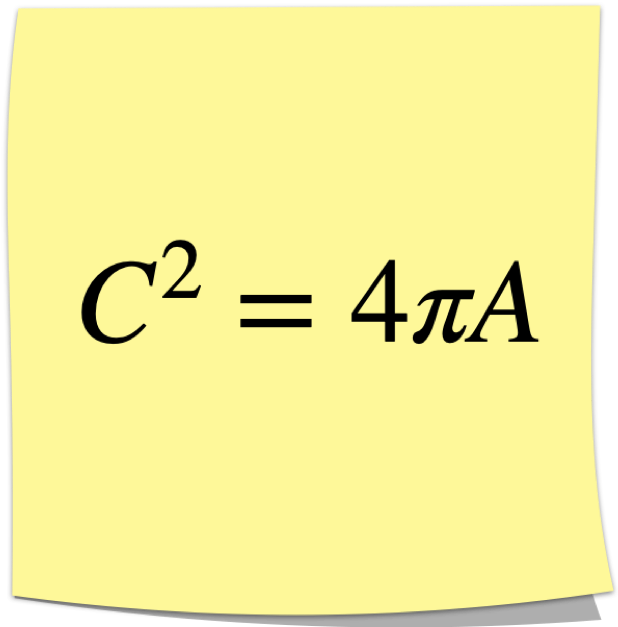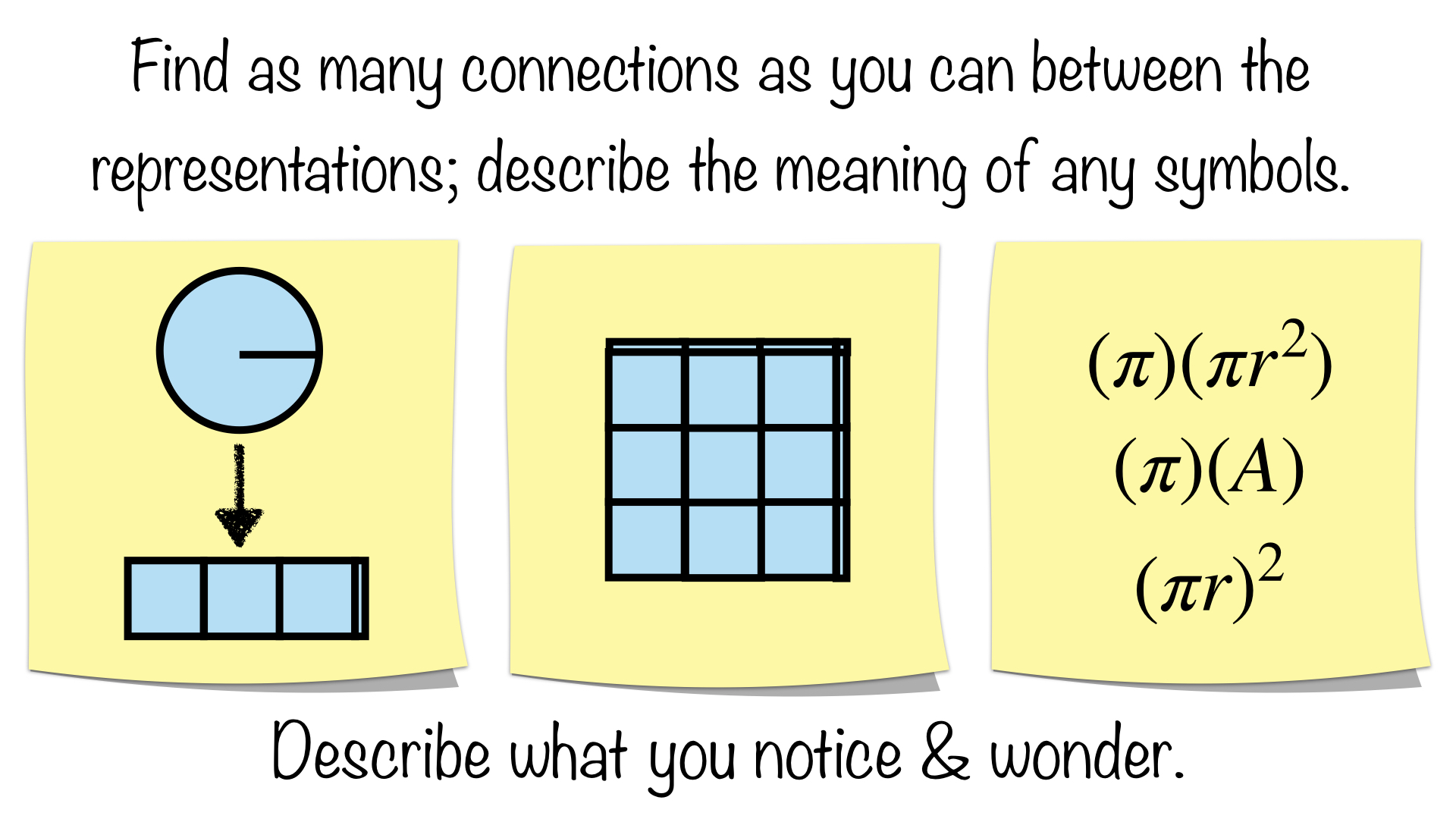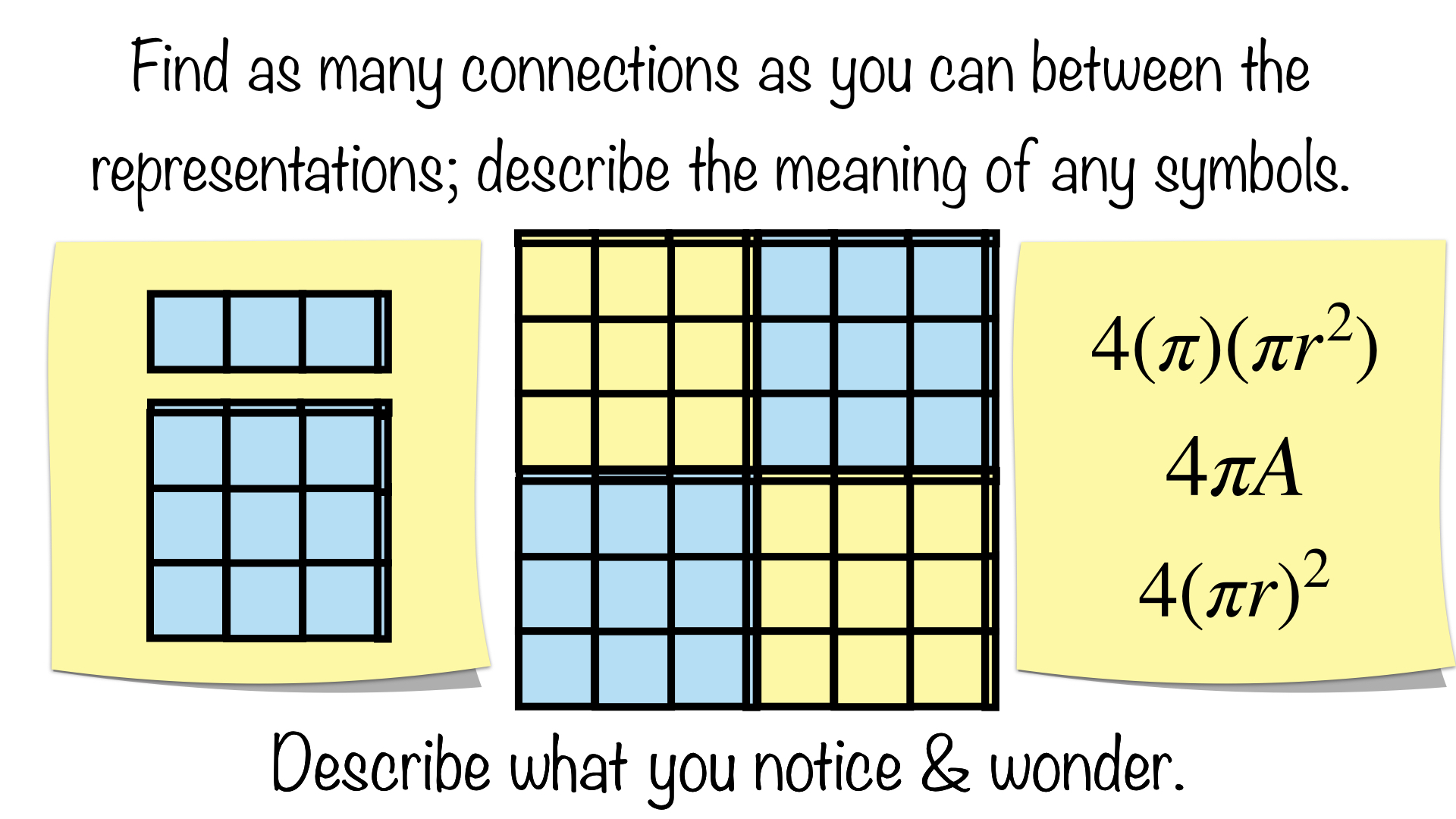Select Page

Common Core Standard: 7.G.B.4

In part one, I provided some Sticky Math Triads to put the process before students and teachers.  While I struggled there to provide visual representations, I believe I have accomplished the goal here as we examine the resulting relationship here in part two.  What follows could accompany part one in a single lesson; although, you might be better off focusing on the manipulation of formulas for one lesson and understanding the relationship in the following lesson.  Both have valuable connections to other concepts you could explore in the process.

WHERE DID WE LEAVE OFF?

Through a series of manipulations, we found that that:What does it mean though?  What is the relationship between the area of circle and its circumference?  The right side is a little more complicated to understand so we will start there.  Let me first acknowledge that you could save a period of instruction by simply telling students the relationship in one of the following ways:

• that squaring a circumference will give you 4π times the radius
• that 4π times the radius will give you the circumference squared
• if you were to square the circumference of a circle, and multiply the area of the same circle by 4π you would get the same value

The problem is that if you did so, then it would be soon forgotten.  In fact, one might argue the value in spending any time on this part of standard 7.G.B.4, “give an informal derivation of the relationship between the circumference and area of a circle.”   I personally cannot think of a single instance of when I had to use this relationship to solve a real word or mathematical problem.  So why spend the time at all?

I would argue that this part of the standard allows us to explore circumference and area in greater depth while allowing students an opportunity to gain and use academic vocabulary; however, besides the process being of value in of itself as illustrated in part one the greatest value of the endeavor might be the exploration of the meaning of a quadratic term, which has multiple applications elsewhere.

With that in mind, we begin with the meaning of the expression on the right side of the equation as it is bit more difficult to envision.

WHAT DOES 4πA LOOK LIKE? BEGINNING WITH A.

Need some rigor for an honors course?  Ask them that question.  The problem is you would not have time to ask them as you would be rushing on to learning more content procedurally.  It is a shame that we limit the beauty and understanding of mathematics through certain pathways of acceleration over others that would not do so.  Common core standards define rigor as conceptual understanding, procedural skills & fluency, and application.  This should be an option for all students; not a select few.  Sticky Math provides the option for all students. So let’s get started by connecting to previous work from understanding the area of a circle in terms of square units from a previous blogpost (this is best done before engaging in this task):Connections could include:

• the middle sticky reorganizes the pizza slices into what could be made into a rectangle through some cutting
• the radius in the left sticky is the side length of the squares in the middle sticky
• the r squared in the right sticky refers to the squares in the middle sticky
• the length of the rectangle in the middle sticky is half of the circumference in the left sticky (Where is it in the equation?)
• the length of the rectangle is the middle sticky is πr in the right sticky (Where is it in the left sticky? What is does πr mean?
• the height of the rectangle in the middle sticky is the radius, which can be found in picture on the left sticky and the second factor in the right sticky (Where is it in the left sticky?  Where is it in the right sticky?)
• the length of the rectangle in the middle sticky is the radius pi times
• there are pi number of r squares in the middle and right stickies.

Students may not have made all the necessary connections when examining the area formula of a circle when relating it to a rectangle/parallelogram the last time (see former blogpost on the subject); this gives them another opportunity to do so.  I will not elaborate much here as I have already done so in that blogpost; however I will point out the goal here is to understand what the A in 4πA means.  Once we know what A looks like then we can explore πA.

WHAT DOES 4πA LOOK LIKE?  πA

Once A has been established, then we can move onto seeking to understand what πA looks like:Connections could include:

• any connections from the previous Sticky Math Triad
• in the left sticky the rectangle and the circle have the same area
• compared to the left sticky, the middle sticky shows the area of a circle pi times whether you view it as pi rows or pi columns (Students may say three here; follow up with a question like, ” Then what is the extra portion above?  Is it really 3?  Engage all students in pairs as to what they feel the height might be.)
• the first expression on the right sticky refers to number of times (first factor) you have pi times r squared (second factor)
• the second expression on the right sticky refers to number of times (first factor) you have the area of the circle (second factor)
• the third expression on the right sticky refers to side lengths of the square, it is pi, r times on each side or r, pi times on each side

Students and adults may struggle with this one as it is rigorous; it requires the application of conceptual understanding and procedural skills together.  The key is to see and understand area as base and height.  The base in this case is the area of a circle reformatted into square units and the height of it is pi.  Some purposeful questions to help students and adults “see it”:

• Where is/are the rectangle(s) in the left sticky in the middle sticky?  How many of them are there?
• In the middle sticky, if the units are radii, then how long is a side of the square?  Is it a square?
• On the right sticky, what are the factors of the top expression?  What do they mean?  How are they illustrated in the middle sticky?
• On the right sticky, what are the factors of the middle expression?  What do they mean?  How are they illustrated in the middle sticky?
• On the right sticky, what are the factors of the bottom expression?  What do they mean?  How are they illustrated in the middle sticky?  (Expanded notation may help here, it is (πr)(πr).

Once students or adults see that the area of the circle has been repeated pi times or that you have pi groups of the area of a circle, then you can move onto examining the final part of the expression.

WHAT DOES 4πA LOOK LIKE?

If you have gotten this far, then completing the expression with the factor of four should be a little easier to see and to grasp:Connections could include:

• any connections from previous Sticky Math Triads
• the left sticky has one area of a circle, then pi areas of a circle
• the middle “sticky” has 4 pi areas of a circle
• on the right sticky, the first expression shows each component: the area of a circle with pi r squared to include the dimensions, pi number of them to make the smaller square, and four to represent four of these squares
• on the right sticky, the second expression shows: the area of the circle as whole, a row or column depending on how you “see it”; it repeated pi times; and four of them.
• on the right sticky the third expression shows four squares with side lengths of πr

The main takeaway here is that the middle “sticky” is a visual representation of 4πA.  Once that is established, then you can compare it to the other side of the equation circumference squared.

WHAT DOES CIRCUMFERENCE SQUARED LOOK LIKE?

Before we can compare one to another, we must establish what circumference squared looks like.  The pink sticky below represents one of the larger rectangular stickies; using these helps keep the drawings here more to scale.Connections could include:

• in the left sticky, circumference has been “straightened” into a linear representation
• in the left sticky, if you take the circumference as a linear representation and “square” it, then you get the green square
• in the left sticky, the green square has a side length of C, the circumference
• C squared in the right sticky is the green square in the left sticky

The main takeaway here is that C squared is the green square. Once that is established, we can finally compare the two sides of the equation and examine the relationship between them.  The moment we have all been waiting for and working towards! (If you made it this far…)

WHAT IS THE RELATIONSHIP BETWEEN THE AREA OF A CIRCLE & ITS CIRCUMFERENCE?

We have finally arrived. One note, I will refer to the left two drawings below as stickies, but they are really drawings made to scale.  This is one of those times, where you probably will not be drawing the representations on a sticky note and projecting it.  Another time is the Pizza Pi stickies above.  Feel free to project them from the gallery.  By the way, the yellow, blue and green are not coincidental.  Remember your primary colors: yellow & blue make green!Connections could include:

• in the left sticky, circumference has been “straightened” into a linear representation and squared
• the C squared in the right sticky is the green square (acting as a left sticky)
• the 4πA in the right sticky is the yellow/blue square (acting as a middle sticky)
• the two large squares, the left and the middle are congruent, which illustrates the equality in the right sticky
• 4π areas take up the same space as the circumference squared
• the left square illustrates the left side of the equation & the right square illustrates the right side of the equation

There you have it; a visual representation of the relationship between the area of a circe and its circumference!  Other representations like verbal descriptions are great formative assessments as whether or not students are “seeing” the relationship correctly, and there is a third sticky above to request students to construct them.  Or, you could use either of the following:So, I feel like this will be the best blogpost that no one will ever read.  I use “best” in the terms of myself taking the understanding of algebra as area to new levels personally.  I’m not that arrogant to assume it is the best blogpost ever; far from it; although, I do try to always acknowledge some level of arrogance.  I hope if you did make it through that it added to your understanding of algebra as area, which you can take before students whether it is in the form of these stickies or others.  For more on the subject of algebra as area, see my sister site, meaning4memory.com.  Should you try these stickies with students, I would love to hear how it went, and how you might modify them to make them more understandable.

Common Core Standards: 7.G.B.4

What connections are your students making?  What modifications are you making to use this with students?  We would love to hear your feedback; please submit a comment below or consider submitting your own Sticky Math activity here.